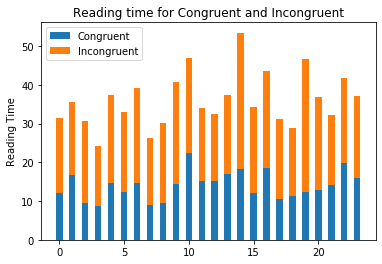# Hypothesis Testing using Stroop Effect

Share this article!

### Analysing the stroop effect

In a Stroop task, participants are presented with a list of words, with each word displayed in a color of ink. The participant’s task is to say out loud the color of the ink in which the word is printed. The task has two conditions: a congruent words condition, and an incongruent words condition. In the congruent words condition, the words being displayed are color words whose names match the colors in which they are printed: for example RED, BLUE. In the incongruent words condition, the words displayed are color words whose names do not match the colors in which they are printed: for example PURPLE, ORANGE. In each case, we measure the time it takes to name the ink colors in equally-sized lists. Each participant will go through and record a time from each condition.

### What is the independent variable? What is the dependent variable?

Independent variable is a variable that is controlled in an experiment. In this problem it is
whether the words are congruent or incongruent.
Dependent variable is the variable being tested. In this problem it is the time to read congruent
and incongruent words

### What is an appropriate set of hypotheses for this task? What kind of statistical test do you expect to perform?

The appropriate hypotheses are if there is a difference between in the reading time of congruent and incongruent words. If there is a difference, we can infer that the Stroop effect exists. correction The null hypotheses is there is no difference between the reading time of congruent words and incongruent words. Mathematically, Ho: u_congruent – u_incongruent = 0 The alternate hypotheses is there is a difference between the reading time of congruent words and incongruent words and Stroop effect does exist. Mathematically, Ha : u_congruent – u_incongruent != 0 where, Ho is null hypotheses, Ha is alternate hypotheses, u_congruent is the population mean of reading time of congruent words, u_incongruent is the population mean of reading time of incongruent words, ‘=’ is arithmetic sign for equal to, ‘!=’ is the sign for not equal to, If we get enough evidence that we can reject null hypotheses, we will support our alternative hypotheses and infer that there is a difference between reading time of congruent and incongruent words i.e. Stroop Effect exist. I’ll perform two side T-test for paired samples. I chose this because the population standard deviation is unknown. The other reason is our two sets of observations are not independent but paired. This means the same person is recorded twice(once for congruent and other for incongruent).

In :
```import pandas as pd
import numpy as np
from scipy import stats
import matplotlib.pyplot as plt
```
In :
```df=pd.read_csv("stroopdata.csv")
```
In :
```df.head()
```
Out:
Congruent Incongruent
0 12.079 19.278
1 16.791 18.741
2 9.564 21.214
3 8.630 15.687
4 14.669 22.803
In :
```df.describe()
```
Out:
Congruent Incongruent
count 24.000000 24.000000
mean 14.051125 22.015917
std 3.559358 4.797057
min 8.630000 15.687000
25% 11.895250 18.716750
50% 14.356500 21.017500
75% 16.200750 24.051500
max 22.328000 35.255000
In :
```array=np.array(df)
```

The visualizations describe the given situations

In :
```N = len(array)
con = array[:,0]
incon = array[:,1]
ind = np.arange(N)
width = 0.5
p1 = plt.bar(ind,con,width)
p2 = plt.bar(ind,incon,width,bottom=con)
plt.ylabel('Reading Time')
plt.title('Reading time for Congruent and Incongruent')
plt.legend((p1, p2), ('Congruent', 'Incongruent'))
plt.show()
```We can clearly see from the visualization that time taken to read incongruent words is higher
than the time taken to read incongruent words.

Now,we perform the statistical test to find out the confidence level and critical statistic value. And check if we can reject the null hypothesis.

In :
```diff=array[:,0]-array[:,1] #Difference vector of two reading times
diff
```
Out:
```array([ -7.199,  -1.95 , -11.65 ,  -7.057,  -8.134,  -8.64 ,  -9.88 ,
-8.407, -11.361, -11.802,  -2.196,  -3.346,  -2.437,  -3.401,
-17.055, -10.028,  -6.644,  -9.79 ,  -6.081, -21.919, -10.95 ,
-3.727,  -2.348,  -5.153])```
In :
```u_diff=diff.mean() #Mean of the difference vector
u_diff
```
Out:
`-7.964791666666666`
In :
```std_diff=diff.std() #Standard Deviation of the difference
std_diff
```
Out:
`4.762398030222158`
In :
```n=len(diff)
n
```
Out:
`24`
In :
```se_diff=std_diff/np.sqrt(n) #Standard Error
se_diff
```
Out:
`0.9721204271733325`
In :
```t=(u_diff-0)/se_diff #Calculated t-statistic
t
```
Out:
`-8.193215000970776`
In :
```dof=n-1 #Degrees of Freedom
dof
```
Out:
`23`
In :
```critical_t= stats.t.ppf(1-0.025, dof) #Crtical value given apha=0.05
critical_t
```
Out:
`2.0686576104190406`
Mean of differences : u_diff = -7.964 Standard deviation of differences : std_diff = 4.762

Standard Error : 0.9721 Degree of Freedom: dof= n-1= 23 Calculated T statistic: t = 8.193 For apha
= 0.05 , Critical Value is +- 2.0686 p value is nearly 0.000001

Hence, our T-statistic is greater than critical value and falls under the critical region, we reject
our Null Hypotheses. Also the p-value is much less than 0.05, hence there is strong evidence in
support of alternate hypotheses.

```
```

Share this article!